# Energy Stored in a Capacitor Derivation, Formula and Applications

0
127

## Energy stored in a capacitor

The energy stored in a capacitor of capacitance C, with charge and voltage is

The electric energy density (energy per unit volume) in a region with electric field is (1/2) ϵoE2 / 2

## How to calculate the energy stored in a capacitor?

Consider a capacitor of capacitance C, completely uncharged in the beginning. Charging process of capacitor requires the expenditure of energy because while charging a capacitor charge is transferred from the plate at lower potential to plate at higher potential. Now if we start charging the capacitor by transporting a charge dQ from negative plate ti the positive plate then work is done against the potential difference across the plate. If q is the amount of charge on the capacitor at any stage of charging process and φ is the potential difference across the plates of capacitor then magnitude of the potential difference is φ=q/C. Now work dW required to transfer dq is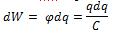To charge the capacitor starting from the uncharged state to some final charge Q work required is Integrating from 0 to Q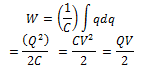which is the energy stored in the capacitor and can also be written as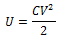we see that the total work done is equal to the average potential V/2 during the charging process, multiplied by the total charge transferred.

If C is measured in Farads, Q in coulombs and V in volts the energy stored would in Joules. A parallel plate capacitor of area A and separation d has capacitance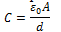Electric field in the space between the plates is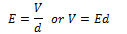Putting above values of V and C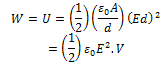If u denotes the energy per unit volume or energy density then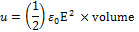The result for above equation is generally valid even for the electrostatic field that is not constant in space.